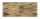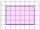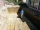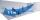# Division + prism - math problems

#### Number of problems found: 12

• Dana helpedDana helped her dad build a sandbox for her younger sister. The sandbox is shaped like a rectangular prism that is 4 1/2 feet long and 4 feet wide. Dana used bags of sand to fill the sandbox 1/2 of a foot deep. Each bag contained 1/2 of a cubic foot of sa
• Waste container ecoThe waste box has the shape of a block with dimensions of 1.8 m, 1.5 m, 1.2 m. 0.25 m3 of waste is added to it every day. How many days will it be filled?
• Cloth / textileWe have cloth measure 16 square meters. How many 20 cm by 20 cm by 8 cm bags you can make? Assume bag is a cuboid without one top base.
• Wooden blockA cuboid-shaped wooden block has 6 cm length, 4 cm breadth, and 1 cm height. Two faces measuring 4 cm x 1 cm are colored in black. Two faces measuring 6 cm x 1 cm are colored in red. Two faces measuring 6 cm x 4 cm are colored in green. The block is divid
• Identical cubesFrom the smallest number of identical cubes whose edge length is expressed by a natural number, can we build a block with dimensions 12dm x 16dm x 20dm?
• The excavationThe excavation for the city sewer was 38 m long, 2.2 m wide, and 3 m deep. a) How many cubic meters of soil were dredged b) How many journeys had to be made by one car when removing the soil if they loaded an average of 4.5 m of cubic soil on each journey
• Cube cutIn the ABCDA'B'C'D'cube, it is guided by the edge of the CC' a plane witch dividing the cube into two perpendicular four-sided and triangular prisms, whose volumes are 3:2. Determine in which ratio the edge AB is divided by this plane.
• Cubes into cuboidHow many 12 centimeter cubes fit into the block (cuboid) with 6dm, 8.4dm, and 4.8?
• Water blockA block with a 50 cm2 base is filled with water 5 cm under the edge. How many can sugar cubes with 2 cm edge be thrown into a container that overflow water?
• GardenGarden with an area of 800 square meters rained 3 mm of water. How many 10-liter cans of water is needed to same irrigation?
• TetrapackHow high should be the milk box in the shape of a prism with base dimensions 8 cm and 8.8 cm if its volume is 1 liter?
• AirRoom is 35.6 m long, 19.6 dm wide and 591 cm high. How many people can simultaneously be in this room if for hygiene reasons is calculated 5000 dm3 of air per person?

We apologize, but in this category are not a lot of examples.
Do you have an exciting math question or word problem that you can't solve? Ask a question or post a math problem, and we can try to solve it.

We will send a solution to your e-mail address. Solved examples are also published here. Please enter the e-mail correctly and check whether you don't have a full mailbox.

Division Problems. Prism Problems.